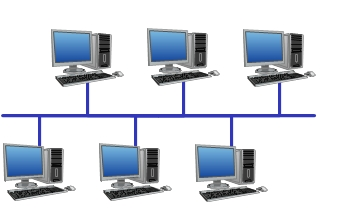# Meaning (Types) Network Topology (Primary 6)

Last Updated on August 26, 2020 by Alabi M. S.

COMPUTER STUDIES

BASIC SCIENCE AND TECHNOLOGY

FIRST TERM

WEEK 4 – NETWORK TOPOLOGY

WEEK 5 – SKETCHING OF NETWORK TOPOLOGY

PRIMARY 6

THEME – BASIC COMPUTER OPERATION

PREVIOUS LESSON – Local Area Network, Metropolitan Area Network and Large or Wide Area Network (Primary 6)

TOPIC: Network Topology

LEARNING AREA

1. Introductory Activities

2. Meaning of Network Topology

3. Types of Network Topology

4. Stretch Different Types of Network Topology

PERFORMANCE OBJECTIVES

By the end of the lesson, the pupils should have attained the following objectives (cognitive, affective and psychomotor):

1. describe network topology;

2. state types of network topology;

3. sketch and describe bus, ring and star topology.

ENTRY BEHAVIOR

Computer System

INSTRUCTIONAL MATERIALS

The teacher will teach the lesson with the aid of –

charts of network topology.

METHOD OF TEACHING – Choose a suitable and appropriate methods for the lessons.

Note – Irrespective of choosing methods of teaching, always introduce an activities that will arouse pupil’s interest or lead them to the lessons.

REFERENCE MATERIALS

Scheme of Work

9 – Years Basic Education Curriculum

Course Book – Tanus Computer Studies book 6

All Relevant Material

Online Information

CONTENT OF THE LESSON

LESSON ONE – INTRODUCTION

Teacher’s/Pupil’s Activities – Displays the chart of different network topology for the pupils to examine and describe.

MEANING OF NETWORK TOPOLOGY

Network topology is the arrangement and description of a computer network.

TYPES OF NETWORK TOPOLOGY

Bus topologyRing topologyStar topologyWEEK 5 – DRAW A BUS, RING AND STAR TOPOLOGY

PRESENTATION

To deliver the lesson, the teacher adopts the following steps:

1. To introduce the lesson, the teacher revises the previous lesson. Based on this, he/she asks the pupils some questions;

2. Leads pupils to define and list types of networking.

Pupil’s Activities – Identify and explain the relationship and the different between network Topology.

3. Teacher’s/Pupil’s Activities – Lesson summary and taking note.

4. Teacher’s/Pupil’s Activities – Draw a bus, ring and star topology.

CONCLUSION

• To conclude the lesson for the week, the teacher revises the entire lesson and links it to the following week’s lesson.

LESSON EVALUATION

Pupils to –

Attempt all the questions.

1. _____________________ is the arrangement and description of a computer network.

A. Computer network

B. Computer system

C. Computer network topology

D. Computer hardware

2. There are ________ types of network topology.

A. 1

B. 2

C. 3

D. 4

3. _____________________ types of network topology looks like a circle.

A. Bus topology

B. Ring topology

C. Star topology

D. Circle topology

4. _____________________ types of network topology looks like a sitting bus.

A. Bus topology

B. Ring topology

C. Star topology

D. Circle topology

5. _____________________ types of network topology looks like a star.

A. Bus topology

B. Ring topology

C. Star topology

D. Circle topology

6. Network topology is the _____________________ and _____________________ of a computer network.

Mention the all the network topology.

7. _____________________

8. _____________________

9. _____________________

10. What is network topology?

WEEK 5 ASSESSMENT

Draw

1. Bus topology

2. Ring topology

3. Star topology

error: